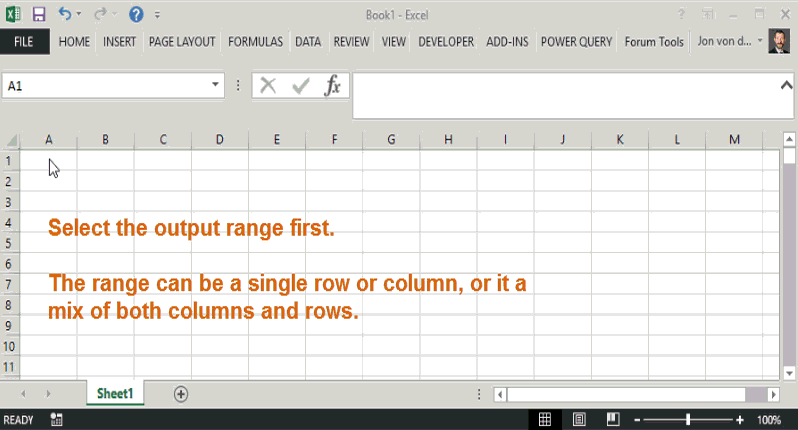# RandBetweenA Function

RandBetweenA is an Excel worksheet function (UDF) that generates unique random numbers between a minimum and maximum number range. This article describes the formula syntax and usage of the worksheet function.

NOTE: This function requires that the VBA code be implemented in the target workbook, or be contained in an addin. If contained in an addin, all parties to whom the code is distributed must also have the addin installed.

# Description

Returns an array of unique random numbers (i.e. each number, although random and unordered, will appear only once). Microsoft’s RAND() function returns random decimal numbers between 0 and 1. RANDBETWEEN() will return random whole numbers within minimum and maxiumum value constraints. RandBetweenA allows you to choose how many decimal places the random number may yield, as well as the minimum and maximum constraints.

# Syntax

RANDBETWEEN(Lower, Upper, [Decimal_Places], [Volatile])

The RandBetweenA function has the following arguments:

• dblLower: Required. The lower bound of the random number. No random number will be less than this argument value.
• dblUpper: Required. The upper bound of the random number. No random number will be greater than this argument value.
• lngDecimals: Optional. A whole number that specifying the bound of the decimal value. The default is zero (i.e. if omitted then RandBetweenA will return whole numbers).
• blnVolatile: Optional. True if you want the random numbers to be recalculated (regenerated) each time calculation is invoked, otherwise False. The default is False.

# Remarks

• RandBetweenA is an array formula and must always be committed with CONTROL + SHIFT + ENTER. If done correctly, Excel will surround the formula in curly brackets {}. Do not attempt to type the curly brackets in.
• The range that RandBetweenA is committed to should be proportionate to the resultant array result, or contain less cells than unique values. For example, if you want random numbers between 1 and 10, then the range it is committed to should not contain more than 10 cells.
• Cells beyond the scope of possible unique random numbers will yield the #NUM! error.

# Example

## Example 1: Basic Demo

Requirement: Generate 10 random numbers. The results must be whole numbers between 1 and 10. The results should be volatile, which means that new numbers should be generated each time recalculation is invoked (e.g. by pressing F9 on the keyboard).## Example 2: Detailed Usage

Requirement: Generate 100 random numbers. The results must be 2-decimal-place numbers between 5 and 5.99. The results should not be volatile, which means that the random number results should persist when recalculation is invoked.